To figure out the probability of the intersection of two events, you use the multiplication rule. This is used to determine the probability that two events are both true.

For example, suppose an experiment consists of choosing a card from a standard deck. Event A = "the card is red." Event B = "the card is a king." The multiplication rule could be used to determine the probability that the card is both red and a king (that is, a red king.)

The multiplication rule can be written in two equivalent ways: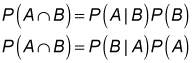Note that these formulas are simply algebraic rearrangements of the definition of conditional probability: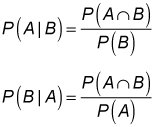Suppose the Omega Corporation has been the subject of takeover rumors for several months. The takeover is far more likely to occur if the economy rebounds next year. Omega's chief economist estimates that the likelihood of strong growth next year is 5 percent, the likelihood of weak growth is 35 percent, and the likelihood of negative growth is 60 percent. The likelihood of a takeover during a period of strong growth is estimated to be 40 percent; during a period of weak growth, this falls to 20 percent; and during a period of negative growth, it's assumed to be only 5 percent. What is the probability that there is strong growth next year and Omega is taken over?

The following events are defined:

• S = "strong growth"

• W = "weak growth"

• N = "negative growth"

• T = "Omega is taken over"

The probability of the events S and T can be determined as follows: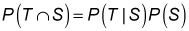Because there's a 5 percent chance of strong growth next year, P(S) = 0.05. The likelihood of a takeover during a period of strong growth is estimated to be 40 percent. Therefore, P(T|S) = 0.40. So the probability that there's strong growth next year and that Omega is taken over is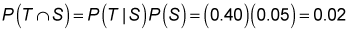When two events A and B are independent, the multiplication rule simplifies to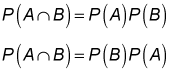This is because P(A|B) = P(A) and P(B|A) = P(B).